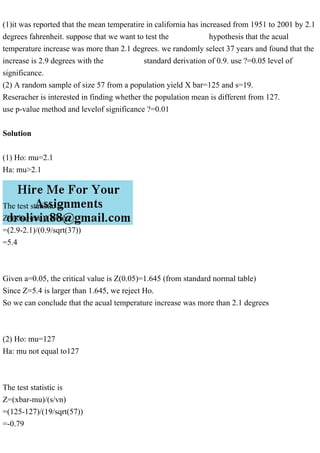PublicitéProchain SlideShare(1). A buiding has two independent security alarm systems. The first.pdf
Chargement dans ... 3
1 sur 2
Publicité

### (1)it was reported that the mean temperatire in california has incre.pdf

1. (1)it was reported that the mean temperatire in california has increased from 1951 to 2001 by 2.1 degrees fahrenheit. suppose that we want to test the hypothesis that the acual temperature increase was more than 2.1 degrees. we randomly select 37 years and found that the increase is 2.9 degrees with the standard derivation of 0.9. use ?=0.05 level of significance. (2) A random sample of size 57 from a population yield X bar=125 and s=19. Reseracher is interested in finding whether the population mean is different from 127. use p-value method and levelof significance ?=0.01 Solution (1) Ho: mu=2.1 Ha: mu>2.1 The test statistic is Z=(xbar-mu)/(s/vn) =(2.9-2.1)/(0.9/sqrt(37)) =5.4 Given a=0.05, the critical value is Z(0.05)=1.645 (from standard normal table) Since Z=5.4 is larger than 1.645, we reject Ho. So we can conclude that the acual temperature increase was more than 2.1 degrees (2) Ho: mu=127 Ha: mu not equal to127 The test statistic is Z=(xbar-mu)/(s/vn) =(125-127)/(19/sqrt(57)) =-0.79
2. The p-value= 2*P(Z<-0.79) =0.4295 (from standard normal table) Since the p-value is larger than 0.01, we do not reject Ho. So we can not conclude thatthe population mean is different from 127
Publicité